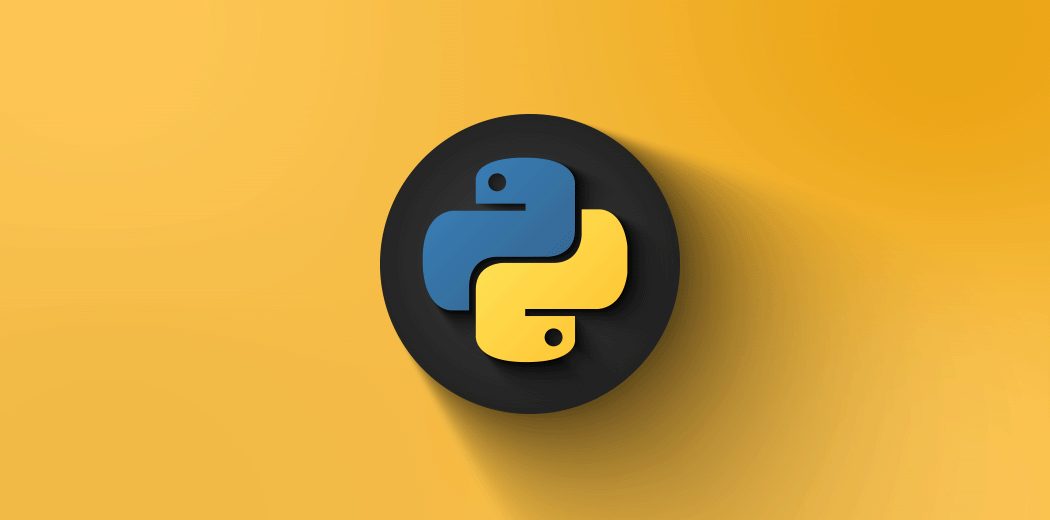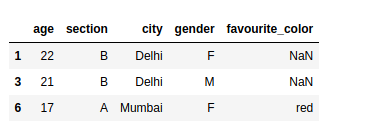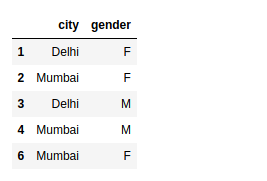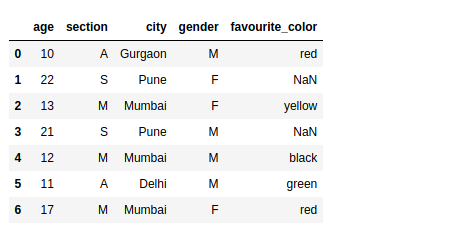LAKSHAY ARORA — February 14, 2020

## What is the difference between loc and iloc in Pandas?

Put this down as one of the most common questions you’ll hear from Python newcomers and data science aspirants. There is a high probability you’ll encounter this question in a data scientist or data analyst interview.

Honestly, even I was confused initially when I started learning Python a few years back. But don’t worry! loc vs. iloc in Pandas might be a tricky question – but the answer is quite simple once you get the hang of it.And that’s what I aim to help you achieve in this article. We will rely on Pandas, the most popular Python library, to answer the loc vs. iloc question.

The Pandas library contains multiple methods for convenient data filtering – loc and iloc among them. Using these, we can do practically any data selection task on Pandas dataframes.

Do check out our two popular Python courses if you’re new to Python programming. They’re free and a great first step in your machine learning journey:

Right, let’s dive in!

## loc vs. iloc in Pandas

So, what is loc and iloc in the first place? We need to answer this question before we can understand where to use each of these Pandas functions in Python.

### loc in Pandas

loc is label-based, which means that we have to specify the name of the rows and columns that we need to filter out.

For example, let’s say we search for the rows whose index is 1, 2 or 100. We will not get the first, second or the hundredth row here. Instead, we will get the results only if the name of any index is 1, 2 or 100.

So, we can filter the data using the loc function in Pandas even if the indices are not an integer in our dataset.

### iloc in Pandas

On the other hand, iloc is integer index-based. So here, we have to specify rows and columns by their integer index.

Let’s say we search for the rows with index 1, 2 or 100. It will return the first, second and hundredth row, regardless of the name or labels we have in the index in our dataset.

We will see multiple examples in this article on how to use loc and iloc for the data selection and data update process in Python.

## loc and iloc in Action (using Pandas in Python)

Time to fire up your Jupyter notebook! We’ll dive straight into the code and understand how and where to use loc vs. iloc in Python.

### Create a sample dataset

First, we need a dataset to apply loc and iloc, right? Let’s do that.

We will create a sample student dataset consisting of 5 columns – age, section, city, gender, and favorite color. This dataset will contain both numerical as well as categorical variables:

### Find all the rows based on any condition in a column

One thing we use almost always when we’re exploring a dataset – filtering the data based on a given condition. For example, we might need to find all the rows in our dataset where age is more than x years, or the city is Delhi, and so on.

We can solve types of queries with a simple line of code using pandas.DataFrame.loc[]. We just need to pass the condition within the loc statement.

Let’s try to find the rows where the value of age is greater than or equal to 15:Try out the above code in the live coding window below!!

### Find all the rows with more than one condition

Similarly, we can also use multiple conditions to filter our data, such as finding all the rows where the age is greater than or equal to 12 and the gender is also male:

### Select a range of rows using loc

Using loc, we can also slice the Pandas dataframe over a range of indices. If the indices are not in the sorted order, it will select only the rows with index 1 and 3 (as you’ll see in the below example). And if the indices are not numbers, then we cannot slice our dataframe.

In that case, we need to use the iloc function to slice our Pandas dataframe.

### Select only required columns with a condition

We can also select the columns that are required of the rows that satisfy our condition.

For example, if our dataset contains hundreds of columns and we want to view only a few of them, then we can add a list of columns after the condition within the loc statement itself:### Update the values of a particular column on selected rows

This is one of my favorite hacks in Python Pandas!

We often have to update values in our dataset based on a certain condition. For example, if the values in age are greater than equal to 12, then we want to update the values of the column section to be “M”.

We can do this by running a for loop as well but if our dataset is big in size, then it would take forever to complete the task. Using loc in Pandas, we can do this within seconds, even on bigger datasets!

We just need to specify the condition followed by the target column and then assign the value with which we want to update:

### Update the values of multiple columns on selected rows

If we want to update multiple columns with different values, then we can use the below syntax.

In this example, if the value in the column age is greater than 20, then the loc function will update the values in the column section with “S” and the values in the column city with Pune:### Select rows with indices using iloc

When we are using iloc, we need to specify the rows and columns by their integer index. If we want to select only the first and third row, we simply need to put this into a list in the iloc statement with our dataframe:### Select rows with particular indices and particular columns

Earlier, we selected a few columns from the dataset using the loc function. We can do this using the iloc function. Keep in mind that we need to provide the index number of the column instead of the column name:

### Select a range of rows using iloc

We can slice a dataframe using iloc as well. We need to provide the start_index and end_index+1 to slice a given dataframe. If the indices are not the sorted numbers even then it will select the starting_index row number up to the end_index:### Select a range of rows and columns using iloc

Slice the data frame over both rows and columns. In the below example, we selected the rows from (1-2) and columns from (2-3).loc and iloc are two super useful functions in Pandas that I’ve come to rely on a lot. I’m sure you’ll be using them as well in your machine learning journey. And if you’re an R user switching to Python, I’m sure you’ll find loc and iloc quite intuitive.

I highly recommend taking our Python for Data Science and Pandas for Data Analysis in Python courses if you’re new to Python programming. They’re free and a great first step in your machine learning journey.###### LAKSHAY ARORA

Ideas have always excited me. The fact that we could dream of something and bring it to reality fascinates me. Computer Science provides me a window to do exactly that. I love programming and use it to solve problems and a beginner in the field of Data Science.